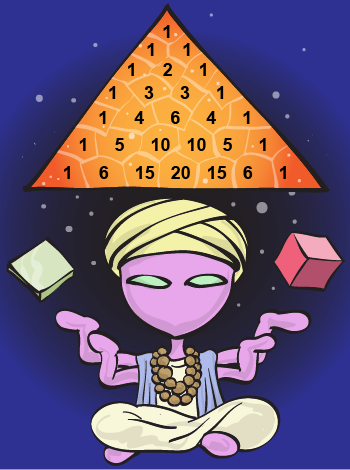Study Guide

# Polynomials

## Polynomials Introduction

Onward, mathronaut; back to the planet of polynomials to search out new life, or at least find our little strange friends to check out all the new things they've learned.

We'll be adding, subtracting, multiplying, and dividing those little guys like we never have before.

We'll discover a new trick: "The Remainder Theorem"—yes, we know it's a strange name. But this guy's amazing. With him, we can factor polynomials, find roots, and even draw squiggly lines (which we'll call graphs).

Our funny little polynomial friends have evolved and can now solve equations. We'll see how they use perfect square trinomials and the difference between two squares, and how a few can even work with cubes. One subspecies, "The Binomial Theorem," even teamed up with Pascal to create Pascal's Triangle. It's sort of like the Bermuda Triangle, but a lot less scary.By the end of it all, we'll have a full grab-bag of tricks to use when working with polynomials. Blast off!

## Polynomials Resources

### Websites

AlgebraLAB: Graphing Polynomials
More help with graphing those pesky polynomials.

Purplemath: Polynomial Graphs, End Behavior
When you're graphing polynomials, it helps to already have an idea of what basic polynomial shapes look like. That way we'll avoid some of the nastier surprises.

Math is Fun: Math Resources
Need more help with the Binomial Theorem? This site makes  a nice Shmoop supplement.

Analyze Math: Graphing Polynomials
Know "zero" about zeros? Check out this site. A tutorial on graphing polynomial functions using the zeros and the x- and y-intercepts.

### Videos

Patrick JMT – The Binomial Theorem
Sometimes we need a step-by-step explanation done in real time. Other times, we need a cookie.

Solve Me Maybe – Parody of Call Me Maybe
A silly song about solving math problems? Yes, we can watch that.

Algebra 2 – Dividing Polynomials using Synthetic Division
Is synthetic division artificially hard you? Watch this and become a natural at it.

### Games and Tools

Jeopardy-Style Algebra
We can hear the Jeopardy theme in our head already. Covers operations, equations, coordinate geometry, data, and probability.